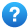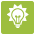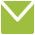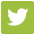#Support Center

## Using the Planting Details Calculator

Last Updated: Feb 20, 2014 05:28PM EST

###If you know how many seeds you will be planting and want to calculate your row and bed length, as well as your expected harvest for a planting, select the Calculating from Number of Seeds option at the top of the Calculator.

You will notice that some of the boxes are shaded in gray.  These boxes are calculated using the AgSquared calculator tool.

As you enter data, the AgSquared Calculators will work for you and calculate the data in the gray shaded boxes based on the information that you have entered in to the white unshaded boxes. The steps are outlined in Red below:In theSection:When Calculating from the Number of seeds, the first step is to enter the number of seeds you intend on planting.The second step is to enter the Within Row Spacing and it’s measurement in the dropdown menu.

Now the AgSquared Calculator will calculate the Total Row Length, based on the Number of seeds and the Within Row Spacing.  You will need to choose a measurement in the dropdown menu.Enter the number of Rows per Bed

Now the AgSquared Calculator will calculate the Bed Length, based on the Total Row Length and the Number of Rows per Bed. You will need to choose a measurement in the dropdown menu.Enter the Bed Width

Now the AgSquared Calculator will calculate the Total Area, based on the Bed Length and Width.  You will need to choose a measurement in the dropdown menu.Enter the Between Row Spacing

You will need to choose a measurement for the Between Row Spacing in the dropdown menu.

In theSection:Enter the Expected yield per row footEnter the measurement per row foot of the Expected Yield

Now the AgSquared Calculator will calculate the Expected Harvest based on the Expected Yield per Row Foot and the total number of Row Feet in the planting.

###When you are Calculating from Expected Harvest, you are calculating from the bottom up.  You are figuring your Row and Bed Space, as well as Number of Seeds based on your Expected Harvest.

You will notice that some of the boxes are shaded in gray.  These boxes are calculated using the AgSquared calculator tool.
You will will need to enter information working from the bottom of the Edit Planting form up to the top.

As you enter data, the AgSquared Calculators will work for you and calculate the data in the gray shaded boxes based on the information that you have entered in to the white unshaded boxes. The steps are outlined in Red below:

Please Note: You are working from the Bottom of the Edit Sowing Details form up to the top when calculating from Expected Harvest.In theSection:Enter the Expected Harvest.  You will need to choose a measurement in the dropdown menu.Enter the measurement per row foot that you will use for the Expected Yield

In theSection:Enter the Expected Yield per row foot

Now the AgSquared Calculator will calculate the Total Row Length, based on the measurement per row foot of the Expected Yield and the Expected Yield.  You will need to choose a measurement in the dropdown menu.Enter the Between Row Spacing.  You will need to choose a measurement for the Between Row Spacing in the dropdown menu.Enter the Bed Width.  You will need to choose a measurement in the dropdown menu.Enter the number of Rows per Bed

Now the AgSquared Calculator will calculate the Bed Length and Total area, based on all of the data that you have entered. You will need to choose measurements in the dropdown menu for the Bed Length and Total area.Enter the Within Row Spacing and it’s measurement in the dropdown menu.

Now the AgSquared Calculator will calculate the Number of seeds required for this planting based on the Total

Calculating From Planting Area for an In-Ground PlantingYou can use the Calculate from Area feature to calculate how many seeds you will need to plant based on how much space you have available in a field, bed, or bench Row and Bed.

You will notice that some of the boxes are shaded in gray.  These boxes are calculated using the AgSquared calculator tool.

As you enter data, the AgSquared Calculators will work for you and calculate the data in the gray shaded boxes based on the information that you have entered in to the white boxes. The steps for Calculating from Area are outlined in Red below:

Please Note: You are working from the Bottom of the Edit Sowing Details form up to the top when calculating from Expected Harvest.In thesection:Enter the Area available for the planting.  You can choose a to calculate from Total Area, Total Row Length or Bed Length.  You will also need to choose a measurement in the drop down menu.Enter the Between Row Spacing.  Please note that this specific data entry field is optional and will not affect your calculations.  You will need to choose a measurement in the dropdown menu.Enter the Width of your Planting Bed.  You will need to choose a measurement in the dropdown menu.Enter the Number of Rows per BedEnter your within row spacing.  This is the space in between each plant in the row.  You will need to choose a measurement in the dropdown menu.  Now the AgSquared Calculator will calculate the Number of seeds required for this planting based on the Planting Area available using all of the information that you have entered into the edit planting form.

thesection:Enter your expected yield and Unit of Measurement per row foot.  Please note that this information is optional and the only calculation that this will affect is the Expected Harvest.

###This method of Editing Sowing details turns off the AgSquared calculator features and allows the user to enter all data into the form.  This leaves a bit more flexibility for growers that have equations which do not fit into the calculator formulas.  This approach also allows the user to fill in some fields while leaving others empty.

It is important to remember that if you use No Calculations to create planting data and then change your Calculate from to Number of Seeds or Expected Seeds any value that you enter will in turn re-calculate all of the related values based on the new value that you have entered.

If at any time you find that you have accidentally shifted the values of your plantings just pressand your planting will revert to the last saved values.

Please Note: Using the No Calculations method works the same when calculating for In-Ground or Container. then In-Ground Plantings

### Calculating From Number of Seeds for a Container, then In-Ground PlantingWhen Calculating from Number of Seeds, you are doing exactly that- Calculating your Row and Bed Length, as well as your Expected Harvest based on the Number of Seeds that you intend to plant.

You will notice that some of the boxes are shaded in gray.  These boxes are calculated using the AgSquared calculator tool.

As you enter data, the AgSquared Calculators will work for you and calculate the data in the gray shaded boxes based on the information that you have entered into the white unshaded boxes. The steps are outlined in Red below:In theSection:Enter the Number of SeedsEnter the Number of Seeds per Container

Now the AgSquared Calculator will calculate Number of containers based on the Total Number of seeds and the number of Seeds per containerEnter the Footprint of the Containers.  The footprint is the amount of space that the container takes up.  The footprint is measured in a squared unit of measurement.  You will need to choose a squared unit of measurement in the dropdown menu.

Now the AgSquared Calculator will calculate the Total Bench space based on the Number of Containers and their footprint.  You will need to choose a squared unit of measurement in the dropdown menu.Enter a Germination Rate.  The Germination rate will determine the total number of transplants, that appears in the- Number of transplants box

In thesection:

Now the AgSquared Calculator will calculate the Number of Transplants based on the Number of Seeds, Number of Containers and the Germination Rate.The next step is to enter the Within Row Spacing and it’s measurement in the dropdown menu.

Now the AgSquared Calculator will calculate the Total Row Length, based on the Number of Transplants and the Within Row Spacing.  You will need to choose a measurement in the dropdown menu.Enter the number of Rows per Bed

Now the AgSquared Calculator will calculate the Bed Length, based on the Total Row Length and the Number of Rows per Bed. You will need to choose a measurement in the dropdown menu.Enter the Bed Width

Now the AgSquared Calculator will calculate the Total Area, based on the Bed Length and Width.  You will need to choose a measurement in the dropdown menu.Enter the Between Row Spacing

You will need to choose a measurement for the Between Row Spacing in the dropdown menu.

In theSection:Enter the Expected yieldEnter the measurement per row foot of the Expected Yield

Now the AgSquared Calculator will calculate the Expected Harvest based on the Expected Yield per Row Foot and the total number of Row Feet in the planting.

### Calculating From Expected Harvest for a Container, then In-Ground PlantingWhen you are Calculating from Expected Harvest, you are calculating from the bottom up.  You are figuring out your Row and Bed Space, as well as Number of Seeds based on your Expected Harvest.

You will notice that some of the boxes are shaded in gray.  These boxes are calculated using the AgSquared calculator tool.
You will will need to enter information from the bottom of the Edit Planting form working up to the top.

As you enter data, the AgSquared Calculators will work for you and calculate the data in the gray shaded boxes based on the information that you have entered in to the white unshaded boxes. The steps are outlined in Red below:

Please Note: You are working from the Bottom of the Edit Sowing Details form up to the top when calculating from Expected Harvest.In theSection:Enter the Expected Harvest.  You will need to choose a measurement in the dropdown menu.Enter the measurement per row foot that you will use for the Expected YieldEnter the Expected Yield per row foot

Now the AgSquared Calculator will calculate the Total Row Length, based on the measurement per row foot of the Expected Yield and the Expected Yield.  You will need to choose a measurement in the dropdown menu.

In thesection:Enter the Between Row Spacing.  You will need to choose a measurement for the Between Row Spacing in the dropdown menu.Enter the Bed Width.  You will need to choose a measurement in the dropdown menu.Enter the number of Rows per Bed

Now the AgSquared Calculator will calculate the Bed Length and Total area, based on all of the data that you have entered. You will need to choose measurements in the dropdown menu for the Bed Length and Total area.Enter the Within Row Spacing and it’s measurement in the dropdown menu.

Now the AgSquared Calculator will calculate the Number of Final Transplants required for this planting based on the Total Row Length and the Within Row Spacing.

In theSection:Enter a Germination rate.

Now the AgSquared Calculator will calculate the Number of seeds based on the Number of transplants and the Germination rateEnter the Footprint of the Containers.  The footprint is the amount of space that the container takes up.  The footprint is measured in a squared unit of measurement.  You will need to choose a squared unit of measurement in the dropdown menu.Enter the Number of Seeds per Container

Now the AgSquared Calculator will calculate theTotal Bench space based on the Number of Containers and their footprint.  You will need to choose a squared unit of measurement in the dropdown menu.

Calculating From Planting Area for a Container, then In-Ground PlantingYou can use the Calculate from Area feature to calculate how many seeds you will need to plant based on how much space you have available in a field, bed, or bench.

You will notice that some of the boxes are shaded in gray.  These boxes are calculated using the AgSquared calculator tool.

As you enter data, the AgSquared Calculators will work for you and calculate the data in the gray shaded boxes based on the information that you have entered in to the white boxes. The steps for Calculating from Area are outlined in Red below:In thesection:Enter the Area available for the planting.  You can choose a to calculate from Total Area, Total Row Length or Bed Length.  You will also need to choose a measurement in the drop down menu.Enter the Between Row Spacing.  Please note that this specific data entry field is optional and will not affect your calculations.  You will need to choose a measurement in the dropdown menu.Enter the Width of your Planting Bed.  You will need to choose a measurement in the dropdown menu.Enter the number of rows in the bed.Enter your within row spacing.  This is the space in between each plant in the row.  You will need to choose a measurement in the dropdown menu.

In thesection:Enter a Transplant Survival rate %.   Now the AgSquared Calculator will calculate the Number of Transplants based on the Number of transplants and the Germination rateEnter the Footprint of the Containers.  The footprint is the amount of space that the container takes up.  The footprint is measured in a squared unit of measurement.  You will need to choose a squared unit of measurement in the dropdown menu.Enter the number of transplants in each container.

In thesection:Enter a Germination rate %.  Now the AgSquared Calculator will calculate the Number of Containers required based on the planting's Germination rateEnter the Footprint of the Containers.  The footprint is the amount of space that the container takes up.  The footprint is measured in a squared unit of measurement.  You will need to choose a squared unit of measurement in the dropdown menu.  The calculator will then give you the total bench space required.Enter the Number of Seeds per Container.  Now the AgSquared Calculator will calculate the Number of seeds required for this planting based on the Planting Area available using all of the information that you have entered into the edit planting form.

In theSection:Enter your expected yield and Unit of Measurement per row foot.  Please note that this information is optional and the only calculation that this will affect is the Expected Harvest.

###Training Webinars

• Watch our training webinars for an in-depth walkthrough on getting started with AgSquared.

###Contact Us

•• Meet Dave, Drew, and Mandy from the AgSquared Customer Success team. They’re ready to answer your questions.

• Email Us

###Follow our Tweets

help@agsquared.com
http://assets1.desk.com/
false
desk